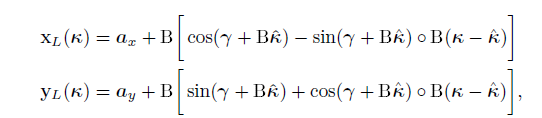# Infeasible Problem Help

  cvx_begin
variable k(n_0,1)
minimize ( norm(D_2*k,1) + 0.2*norm(k,1) )
subject to

cvx_end

Well, apparently your problem is infeasible. You haven’t provided the input data, therefore we can’t diagnose it for you.

You can try solving a problem where, instead of the current objective function, you minimize the LHS of one or both of the constraints (subject, or not, to the other constraint) to determine how close the problem is to feasibility.

Hier is my Problem. I have estimatet k_hat. It is a 751x1 Vector. Why is my Problem infeasible?

Thanks a lota_x = 0; gamma=0;
I have k_hat. It is a 751x1 Vector. The hadamard Product i implemented with .*

You still haven’t provided data to make this a reproducible problem, so I can;t further diagnose it.
IIt appears, however, that you did not properly implement the 2-norm constraint. (5.27) shows a single 2-norm constraint applied to a stacked vector of x^i and y^i for each i. That is not the same as implementing separate 2-norm constraint for x^i and y^i, as you have. Obviously the 2-norm constraint(s) would be feasible with sufficiently large value of \epsilon^i.

Is your N = 1? You’re also missing the constraint on |\kappa|.

My N is 751. ´
The B is a 751x751 Matrix and k_hat is 751x1 Vector.
Do i need a for loop ? Which Data do you need?

Thanks a lot

You an implement the constraints in a for loop.

But really, it is your problem, so you should diagnose why is is not feasible. Obviously, feasibility depends on the input data., certainly to include the values of \epsilon^i. Of course, it appears that you haven’'t gotten the model implemented correctly yet. My comments only highlight some of your mistakes, and don;t necessarily capture all of them, which I I have no way of knowing.

Also note that inearizing constraints, as your extract says was done to produce the expression inside the 2-norm, can be dangerous, and depending on how it is done, could in some cases result in a feasible nonlinear constraint becoming an infeasible linearized constraint. I don’t know whether that can happen in this model.

    subject to
for i=1:n_0